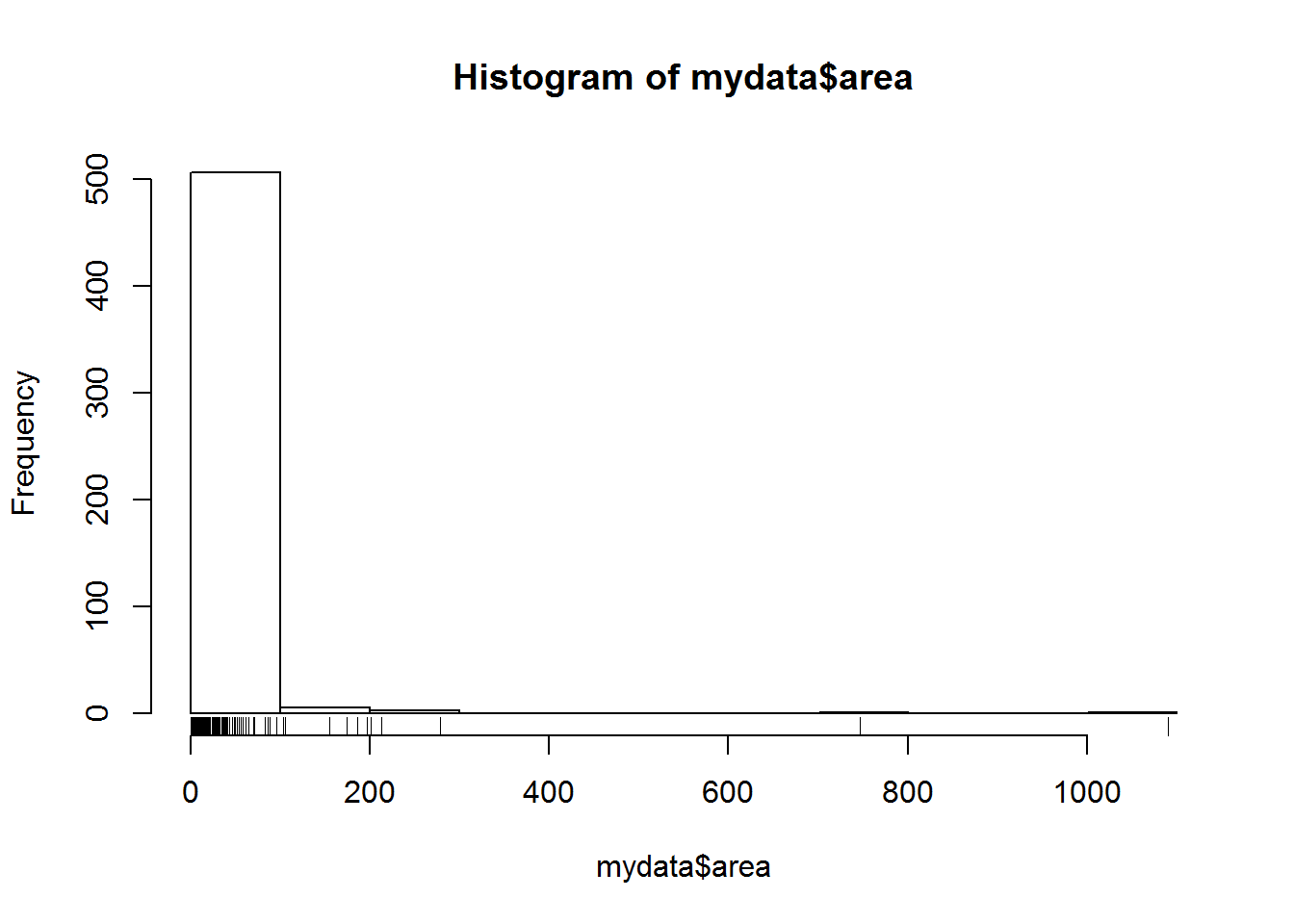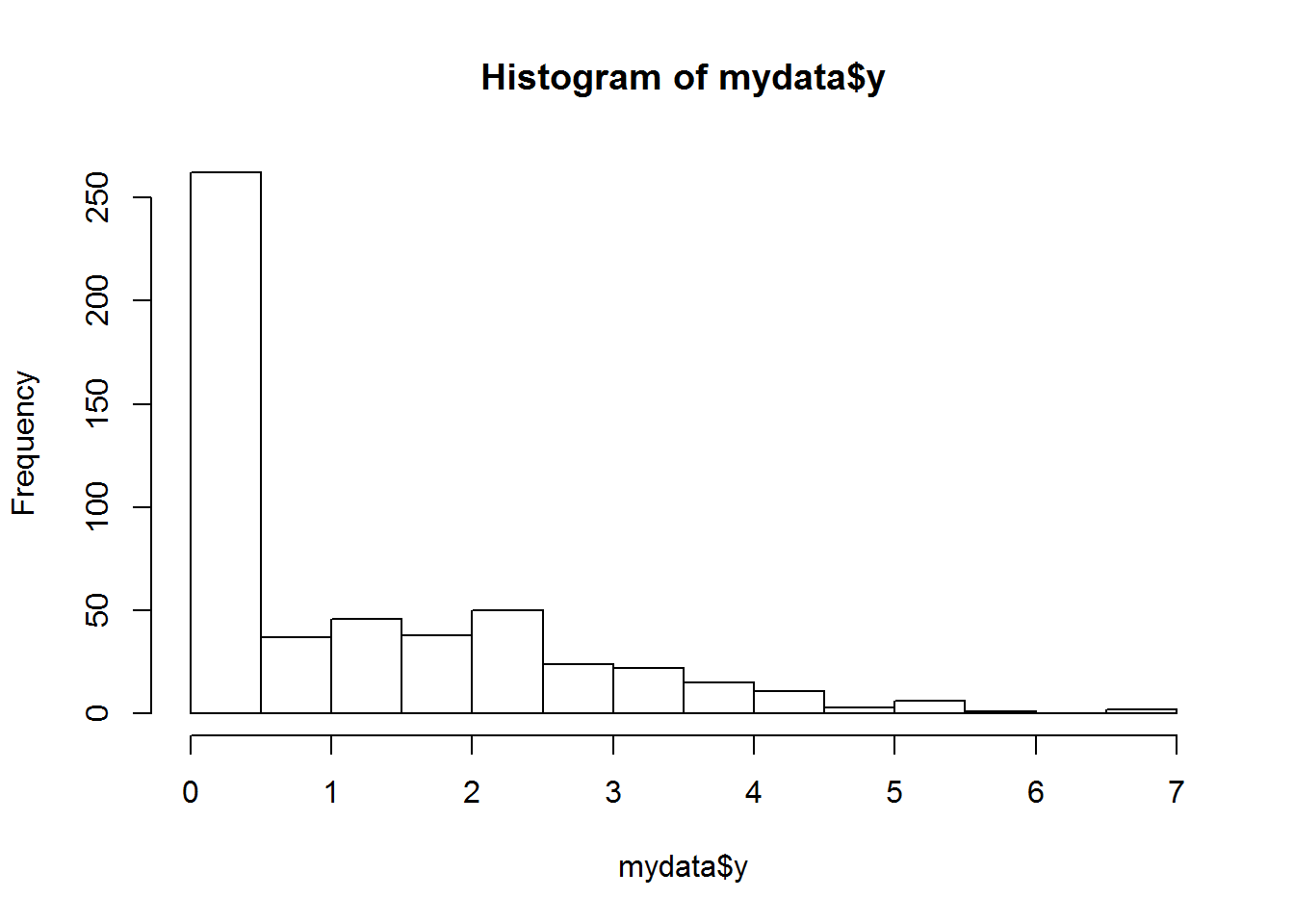# Predicting forest fires using weather data

## Introduction

This post is based on a paper by Cortez & Morais (2007). Forest fires are a major environmental issue, creating economical and ecological damage while endangering human lives. Fast detection is a key element for controlling such phenomenon. To achieve this, one alternative is to use automatic tools based on local sensors, such as microclimate and weather data provided by meteorological stations.

All this data holds valuable information, such as trends and patterns, which can be used to improve decision making. Yet, human experts are limited and may overlook important details. Moreover, classical statistical analysis breaks down when such vast and/or complex data is present. Hence, the alternative is to use automated machine learning tools to analyze the raw data and extract high-level information for the decision-maker.

This is a very difficult regression task. We got the forest fire data from the UCI machine learning repository. Specifically we want to predict the burned area or size of the forest fires in the northeast region of Portugal. We demonstrate the proposed solution of Cortez which includes only four weather variables (i.e. rain, wind, temperature and humidity) in conjunctionwith a support vector machines (SVM) and it is capable of predicting the burned area of small fires, which constitute the majority of the fire occurrences.

A SVM uses a nonlinear mapping to transform the original training data into a higher dimension. Within this new dimension, it searches for the linear optimal separating hyperplane.

## Objective

• Perform a support vector regression.
• Assess the accuracy of the model.
• Demonstrate the work flow for fitting a SVM for classification in R.

## The data

Upon glimpse()ing the data, we notice the area of the burn has a lot of zeroes. We investigate further with a histogram. We may want to log(area+1) transform the area due to the heavy skew and many zeroes (fires that burnt less than a hectare). The variables are fully explained in the original paper.

hist(mydata$area) rug(mydata$area)We transform area into the new response variable y, this would be useful if we wanted to use the SVM for regression.

mydata <- mutate(mydata, y = log(area + 1))  # default is to the base e, y is lower case
hist(mydata$y)We start at an advantage, as we know what model structure for the SVM was most effective for prediction based on the findings of the paper. Thus we can limit our data preparation to a few variables.The proposed solution, which is based in a SVM and requires only four direct weather inputs (i.e. temperature, rain, relative humidity and wind speed) is capable of predicting small fires, which constitute the majority of the fire occurrences. We also need to normalise the continuous variables between zero and one to control for different ranges. However, the function we use does this for us! We show what it would look like if we wanted to set this up manually but we don’t evaluate it. normalise <- function(x) { return((x - min(x)) / (max(x) - min(x))) # subtract the min value in x and divide by the range of values in x. } mydata$temp <- normalise(mydata$temp) mydata$rain <- normalise(mydata$rain) mydata$RH <- normalise(mydata$RH) mydata$wind <- normalise(mydata$wind) ## Classification SVM is better suited to a classification problem. Let’s pretend we’re interested in the weather conditions that give rise to small fires (arbitary set to < 5 hectares), compared to larger fires. Can we classify the type of fire we might expect to see if we send a fireman out with remote meterological data? This may help them bring the right gear. # note, our earlier transformation was redundant,$area gives the same results
sum(mydata$area < 5) # ln(0 + 1) = 0 ##  366 sum(mydata$area >= 5)
##  151

These fires are split unevenly.

mydata$size <- NULL mydata$size <- factor(ifelse(mydata\$area < 5, 1, 0),
labels = c("small", "large"))
#tail(mydata[, c("size", "area")])  #  checks out

## Splitting the data

As usual, we need a training and testing data set to assess how well the model predicts data it hasn’t seen before.

train <- sample(x = nrow(mydata), size = 400, replace = FALSE)  # sample takes place from 1:x, convenience
#test, not train, use - selection

## Method

We use the kernlab package and the ksvm() function therein to fit an SVM using a non-linear kernel. We can use the argument kernel = "polydot" to set it to polynomial or "rbfdot" for a radial basis and "tanhdot" for the complicated sounding hyperbolic tangentsigmoid. Note the hugh amount of parameter customisation that is possible at this stage. For simplicity we use the default settings which will be far from optimal.

m.poly <- ksvm(size ~ temp + RH + wind + rain,
data = mydata[train, ],
kernel = "polydot", C = 1)
##  Setting default kernel parameters
m.poly
## Support Vector Machine object of class "ksvm"
##
## SV type: C-svc  (classification)
##  parameter : cost C = 1
##
## Polynomial kernel function.
##  Hyperparameters : degree =  1  scale =  1  offset =  1
##
## Number of Support Vectors : 245
##
## Objective Function Value : -228.9428
## Training error : 0.285
m.rad <- ksvm(size ~ temp + RH + wind + rain,
data = mydata[train, ],
kernel = "rbfdot", C = 1)
m.rad
## Support Vector Machine object of class "ksvm"
##
## SV type: C-svc  (classification)
##  parameter : cost C = 1
##
## Gaussian Radial Basis kernel function.
##  Hyperparameter : sigma =  0.442405155947824
##
## Number of Support Vectors : 266
##
## Objective Function Value : -222.7114
## Training error : 0.275
m.tan <- ksvm(size ~ temp + RH + wind + rain,
data = mydata[train, ],
kernel = "tanhdot", C = 1)
##  Setting default kernel parameters
m.tan
## Support Vector Machine object of class "ksvm"
##
## SV type: C-svc  (classification)
##  parameter : cost C = 1
##
## Hyperbolic Tangent kernel function.
##  Hyperparameters : scale =  1  offset =  1
##
## Number of Support Vectors : 186
##
## Objective Function Value : -1712.614
## Training error : 0.455

Using the simple defaults, the radial basis non-linear mapping for the SVM appears equivalent to the polynomial, based on the lower training error; with the polynomial slightly better. We should evaulate the model performance using the predict() function. In order to examine how well our classifier performed we need to compare our predicted size of the fire with the actual size in the test dataset.

## Test with training data

pred <- predict(m.rad, newdata = mydata[-train, ], type = "response")

table(pred, mydata[-train, "size"][])  #  [[]] gives the contents of a list
##
## pred    small large
##   small     2     0
##   large    34    81
confusionMatrix(table(pred, mydata[-train, "size"][]), positive = "small")  # from the caret package, also need e1071 package
## Confusion Matrix and Statistics
##
##
## pred    small large
##   small     2     0
##   large    34    81
##
##                Accuracy : 0.7094
##                  95% CI : (0.6183, 0.7896)
##     No Information Rate : 0.6923
##     P-Value [Acc > NIR] : 0.3865
##
##                   Kappa : 0.0753
##  Mcnemar's Test P-Value : 1.519e-08
##
##             Sensitivity : 0.05556
##             Specificity : 1.00000
##          Pos Pred Value : 1.00000
##          Neg Pred Value : 0.70435
##              Prevalence : 0.30769
##          Detection Rate : 0.01709
##    Detection Prevalence : 0.01709
##       Balanced Accuracy : 0.52778
##
##        'Positive' Class : small
## 

## Conclusion

A basic introduciton to SVM in R showing the workflow. Bear in mind we have some way to go in optimising and validating this model! Changing parameters is likely to improve our 70% accuracy achieved with the default settings.